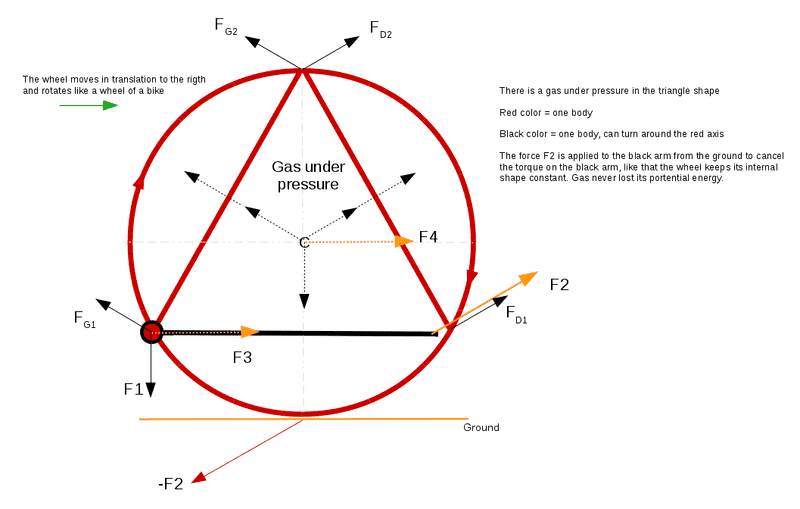# Calculate the forces and the work

## Homework Statement

A wheel moves to the rigth and rotates like a wheel of a bike. There are 2 bodies:

- the wheel (red circle) + 2 red walls
- the black arm

The ground is fixed.

The black arm can rotate around the red axis. The red axis is fixed to the wheel. There is no friction.

The equilateral triangle shape (2 red walls + black arm) contains a gas at pressure P. The pressure P is constant because the surface of the triangle is constant. The device has a thickness of 1 m so I studied it like a 2d problem. There is a gasket between the black arm the and wheel.

The black arm receives from the ground the force F2 (look the image). This force must cancel the torque on the black arm to keep constant the surface of the triangle.

The pressure of the gas is 1 Pa (the pressure outside the triangle is 0)
The thickness is 1 m
The radius of the wheel is 1 m

Questions:

1/ Draw all forces. The sum of forces is on the center of the wheel.
2/ Find the values of the force F2 to apply for cancel the torque on the black arm. Find the values of all forces.
3/ Calculate the sum of energy for a small angle δ, like 0.001 rd for example.

F=p*A
T=F*d
W=F*dl

## The Attempt at a Solution

1/

The forces Fg1 and Fg2 come from the pressure on the left red wall
The forces Fd1 and Fd2 come from the pressure on the right red wall
The force F1 come from the pressure on the black arm
The force F2 come from the ground (the ground receives -F2)
The force F3 come from the force F2 less one force like F1
The force F4 come from the force F3
I drawn in dotted lines all the forces Fd1+Fd2+Fg1+Fg2+F1+F4 in the centerIs it correct ?

2/

The length of one wall (of the triangle) is √3 m so the force on each wall √3 N because the pressure is at 1 Pa and the thickness at 1 m . So, F1=Fd1=Fd2=Fg1=Fg2=√3/2 N.

The torque on the black arm around the red axis is ∫x dx from 0 to √3, it is 1.5 Nm. So the force F2 = 1.5/√3*2 = √3 N

F3 = F4 = F2*cos(π/6) = 1.5 N

Is it correct ?

3/

The moments on the red axis lost an energy:

W1 = (F1*R1+F2*R2)*δ = ( F1*√3/2 + F3*1/2 ) δ = ( √3/2*√3/2 + 1.5/2 ) *δ = 1.5e-3 J

The force F4 on the center gives an energy:

W2 = F*d = F4*R*δ = 1.5e-3 J

The force F2 needs an energy:

The trajectory of the cycloid is :

x=θ-sinθ
y=1-cosθ

The length increases:

dl = ( √ ( (θ-sinθ)² + (1-cos(θ))²) - √ ( ((θ+δ)-sin(θ+δ))² + (1-cos(θ+δ))²) )

with θ at 300°

The energy for the force F2 is at dl*F2 = 4.27e-4 * √3 = 7.39 e-4 J

I don't find the sum of energy at 0. The vertical force works so few that I don't consider it, I forgot a force ?

Simon Bridge
Homework Helper
How are you thinking this contraption will work? What is it expected to do?
BTW: this is so incorrect that it is not worth going into. Scrap it and start again - start with what you hope to achieve.

Dale
Mentor
2020 Award
@Gh778

This is insane. I don't know what is your fascination with overly complicated drawings.

The walls are accelerating so Fg1 is not equal to Fg2 and Fd1 is not equal to Fd2. The ground does not interact with the black arm so F2 does not exist. The black arm is accelerating so "The force F3 come from the force F2 less one force like F1" is not true even after you delete the part about F2. The gasket exerts a force which you have not included. F4 doesn't exist.

Despite all of the myriad forces you drew your system has only 2 degrees of freedom: the distance that it rolls along the ground, and the angle of the hinge for the black bar. The potential energy is only a function of the angle of the hinge, so the Lagrangian is fairly simple. You should analyze it via the Lagrangian. An increase in the angle of the hinge will decrease the potential energy of the gas and increase the potential energy of the gasket. The equilibrium is the point where the rate of increase in the PE of the gasket equals the rate of decrease in the PE of the gas.

To me this looks like a veiled attempt to discuss a perpetual motion machine, so this thread is closed.

Last edited: